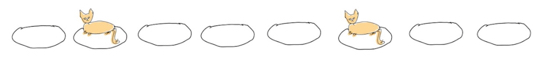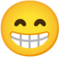## Cats on Cushions Puzzle

Visible to anyone in the world
Edited by Richard Walker, Friday, 13 Nov 2020, 02:15

This is a sawn-down version of a puzzle "Arranging cats and dogs" that Matt Parker recently posted on YouTube.

In our version we have a pair of cats, and eight cushions. We want to seat each cat on its own cushion, with the restriction that they cannot occupy adjacent cushions, in case they start a cat fight. Here is one possible arrangement.You see the cats are not next to one another, so the rule is satisfied.

The question is: how many possible arrangements are there? What if there were 9 cushions? Or 10? Can you give a general formula?

Share post

### New comment

The cats will decide for themselves where they sit, and take no notice of any rules you may set themThey may even just walk awayJan

### New comment

I see you know cats well 😄

### New comment

I looked at it as being an n-bit binary number where only two bits are set and they can't be next to each other. So taking for example 5 cushions (cats)there are 6 possibilities:

1 0 1 0 0
1 0 0 1 0
1 0 0 0 1
0 1 0 1 0
0 1 0 0 1
0 0 1 0 1

So it looks like cat A can occupy all except the last 2 cushions, and for the ones it can occupy, there's a decrease of 1 in the number that cat B can occupy for every rightwards shift (A and B are interchangeable of course, so that A 0 B is the same as B 0 A).

Which I think gives us sigma_thingy(1 - (n-2)) (sorry, don't know how to do the symbol, or the notation, really, but basically it's the sum of all the integers from 1 to n-2)

for the most basic case of 3 where's there's only one configuration (1 0 1) that works out as

sigma 1 - (n-2 = 1) = 1

and for 5, as above:

sigma 1 - (5-2 = 3) = 1 + 2 + 3 = 6

...although as usual, even if that's right, I'm sure there's a simpler and blindingly obvious way

### New comment

That’s right.

The way I saw it, if we call the cats cat1 and cat2 going from left to right, and the cushions are numbered 1, 2, 3... then

If cat1 is at 1 there are n - 2 cushions cat2 can be on

If cat1 is at 2 there are n - 3 cushions cat2 can be on

If cat1 is at 3 there are n - 4 cushions cat2 can be on

...

If cat1 is at n - 2 there is 1 cushion cat2 can be on

So the number of possibilities is

(n - 2) + (n - 3) + (n - 4) + ... + 2 + 1

which amounts to (n - 1) x  (n - 2) / 2. One way to see this is to call the sum S

S = (n - 2) + (n - 3) + (n - 4) + ... + 2 + 1

Writing this backwards and then adding the original equation

S = (n - 2) + (n - 3) + (n - 4) + ... + 2 + 1

S = 1 + 2 + 3 + ... + (n - 3) + (n - 2)

we get (n - 1) repeated (n - 2) times, hence

2S = (n - 1) x (n - 2) and so S = (n - 1) x  (n - 2) / 2. This is a well-known formula.